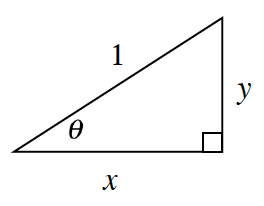### Home > CCG > Chapter 12 > Lesson 12.1.2 > Problem12-30

12-30.Examine the diagram at right.

1. Explain why $y=\sin\theta$ and $x=\cos\theta$.

Use the diagram to write $\sin\theta=$ ?

2. According to this diagram, what is $\left(\sin\theta\right)^2+\left(\cos\theta\right)^2$? Explain how you know.

3. Does this relationship appear true for all angles? Use your calculator to find $\left(\sin23º\right)^2+\left(\cos23º\right)^2$ and $\left(\sin81º\right)^2+\left(\cos81º\right)^2$. Write down your findings.